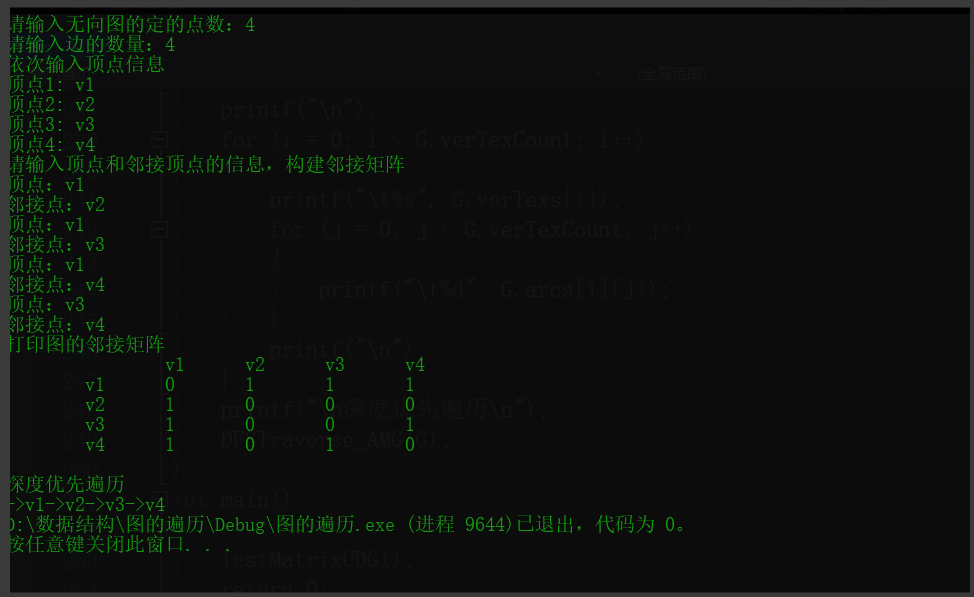## 【图的深度优先遍历C语言版】_Camellia——的博客-程序员ITS304

#define _CRT_SECURE_NO_WARNINGS
#include <stdio.h>
#include<string.h>
#include<stdlib.h>
#define OK 1
#define ERROR 0
#define MAX_VERTEX 100
#define VISITED 1
#define UNVISITED 0
//图的类型枚举
typedef enum
{
DG,//有向图
UDG,//无向图
DN,//有向网
UDN//无向网
}Graphkind;
//设置顶点的数据类型为字符串型-使用时记得
typedef char* VerTexType;
//设置权值类型为整数类型
typedef int ArcType;
//返回的状态类型
typedef int Status;
//保存每个顶点的访问状态 0-未访问 1-已访问
int visitdfs[MAX_VERTEX];
//图的邻接矩阵存储表示
typedef struct
{
VerTexType verTexs[MAX_VERTEX];//顶点数组
ArcType arcs[MAX_VERTEX][MAX_VERTEX];//邻接矩阵（权数组）
int verTexCount;//图的顶点数
int arcCount;//图的边数/弧的数
Graphkind kind;//图的类型
}MathrixGraph;
//返回某个顶点在顶点集合中的下标（从0开始），不存在返回-1
int LocateVex(MathrixGraph* G, VerTexType vex)
{
int index = 0;
while (index < G->verTexCount)
{
if (strcmp(G->verTexs[index], vex) == 0)
break;
index++;
}
return index == G->verTexCount ? -1 : index;
}
//使用邻接矩阵表示法创建无向图
/*

1.无向图的邻接矩阵是对称的
2.顶点的度=第一行（列）中1的个数
*/
Status CreateUDG(MathrixGraph* G)
{
G->kind = UDG;//设置当前创建图的类型为无向图
printf("请输入无向图的定的点数：");
scanf("%d", &G->verTexCount);
printf("请输入边的数量：");
scanf("%d", &G->arcCount);
printf("依次输入顶点信息\n");
int i;
int j;
for (i = 0; i < G->verTexCount; i++)
{
G->verTexs[i] = (VerTexType)malloc(sizeof(char) * 10);
printf("顶点%d: ",i+1);
scanf("%s", G->verTexs[i]);
}
//初始化邻接矩阵，所有边的权值设置为0
for (i = 0; i < G->verTexCount; i++)
{
for (j = 0; j < G->verTexCount; j++)
{
G->arcs[i][j] = 0;
}
}
printf("请输入顶点和邻接顶点的信息，构建邻接矩阵\n");
for (i = 0; i < G->arcCount ; i++)
{
VerTexType vex1 = (VerTexType)malloc(sizeof(char) * 10);
VerTexType vex2 = (VerTexType)malloc(sizeof(char) * 10);
printf("顶点：");
scanf("%s", vex1);
printf("邻接点：");
scanf("%s", vex2);
//分别获得两个顶点在顶点数组中的坐标
int x = LocateVex(G, vex1);
int y = LocateVex(G, vex2);
if (x == -1 || y == -1)
return ERROR;
G->arcs[x][y] = 1;
G->arcs[y][x] = G->arcs[x][y];
free(vex1);
free(vex2);
}
return OK;
}
//使用邻接矩阵表示法创建有向图
/*

1.有向图的邻接矩阵有可能是不对称的
2.顶点的出度=第i行元素之和；顶点的入度=第i列元素之和
3.顶点的度=第i行元素之和+第i列元素之和
*/
Status CreateDG(MathrixGraph* G)
{
G->kind = DG;//设置当前创建图的类型为有向图
printf("请输入有向图的定的点数：");
scanf("%d", &G->verTexCount);
printf("请输入边的数量：");
scanf("%d", &G->arcCount);
printf("依次输入顶点信息\n");
int i;
int j;
for (i = 0; i < G->verTexCount; i++)
{
G->verTexs[i] = (VerTexType)malloc(sizeof(char) * 10);
printf("顶点%d: ", i+1);
scanf("%s", G->verTexs[i]);
}
//初始化邻接矩阵，所有边的权值设置为0
for (i = 0; i < G->verTexCount; i++)
{
for (j = 0; j < G->verTexCount; j++)
{
G->arcs[i][j] = 0;
}
}
printf("请输入顶点和邻接顶点的信息，构建邻接矩阵\n");
for (i = 0; i < G->arcCount; i++)
{
VerTexType vex1 = (VerTexType)malloc(sizeof(char) * 10);
VerTexType vex2 = (VerTexType)malloc(sizeof(char) * 10);
printf("顶点：");
scanf("%s", vex1);
printf("邻接点：");
scanf("%s", vex2);
//分别获得两个顶点在顶点数组中的坐标
int x = LocateVex(G, vex1);
int y = LocateVex(G, vex2);
if (x == -1 || y == -1)
return ERROR;
G->arcs[x][y] = 1;
//G->arcs[y][x] = G->arcs[x][y];//有向图的邻接矩阵有可能不对称
free(vex1);
free(vex2);
}
}
//邻接矩阵深度优先遍历
void DFSTraverse_AMG(MathrixGraph G)
{
void DFS_AMG(MathrixGraph G, int index);
//初始化状态数组
int i = 0;
for (i = 0; i < G.verTexCount; i++)
{
visitdfs[i] = UNVISITED;//初始状态设置为未访问
}
//DFS遍历
for (i = 0; i < G.verTexCount; i++)
{
if (!visitdfs[i])//如果某个顶点未访问
{
//调用遍历函数
DFS_AMG(G, i);
}
}
}
//深度优先搜索的核心算法，index为深度搜索的某个顶点下标
void DFS_AMG(MathrixGraph G,int index)
{
printf("->%s", G.verTexs[index]);//访问当前顶点
visitdfs[index] = VISITED;//更改当前顶点的访问状态
int i;
for (i = FirstAdjMAXVex_AMG(G, G.verTexs[index]); i; i= SecondjVex_AMG(G,G.verTexs[index],G.verTexs[i]))
{
if (!visitdfs[i])
{
DFS_AMG(G, i);//如果没有访问过就继续递归调用搜索
}
}
}
//返回顶点vex所在行中第一个邻接点的下标
{
int i = LocateVex(&G, vex);//找到顶点vex在顶点数组中的下标
if (i == -1)
return ERROR;
int defaultWeight;//默认权重
defaultWeight = G.kind <= 1 ? 0 : INT_MAX;//图/网
//搜索图的邻接矩阵中与顶点vex的第一个邻接点的下标
for (int j = 0; j < G.verTexCount; j++)
{
if (G.arcs[i][j] != defaultWeight)
{
return j;
}
}
return 0;
}
//返回与顶点vex1邻接的另一个邻接点（除vex2）的下一个邻接点，没有就返回-1
int SecondjVex_AMG(MathrixGraph G, VerTexType vex1, VerTexType vex2)
{
int index1 = LocateVex(&G, vex1);
int index2 = LocateVex(&G, vex2);
if (index1 == -1 || index2 == -1)
return -1;
int defaultWeight;
defaultWeight = G.kind <= 1 ? 0 : INT_MAX;
for (int i = index2 + 1; i < G.verTexCount; i++)
{
if (G.arcs[index1][i] != defaultWeight)
{
return i;
}
}
return 0;
}
void TestMatrixUDG()
{
int i;
int j;
MathrixGraph G;
//创建无向图
Status status = CreateUDG(&G);
//创建有向图
//Status status = CreateDG(&G);
if (status == ERROR)
{
printf("创建图失败，请检查后重试\n");
}
printf("打印图的邻接矩阵\n");
printf("\t");
for (i = 0; i < G.verTexCount; i++)
{
printf("\t%s", G.verTexs[i]);
}
printf("\n");
for (i = 0; i < G.verTexCount; i++)
{
printf("\t%s", G.verTexs[i]);
for (j = 0; j < G.verTexCount; j++)
{
printf("\t%d", G.arcs[i][j]);
}
printf("\n");
}
printf("\n深度优先遍历\n");
DFSTraverse_AMG(G);
}
int main()
{
TestMatrixUDG();
return 0;
}### JDBC学习_無言46的博客-程序员ITS304

JDBC学习这里写目录标题JDBC学习一级目录二级目录三级目录一、JDBC开发的六个步骤二、SQL注入问题（Statement与preparement)1、Statement 的sql注入问题2、使用PrepareStatement解决sql注入问题三、ORM对象关系映射四、JDBC工具类1、数据源写到dp.properties文件中2、工具类的封装五、三层架构六、JDBC事务处理转账问题1、DAO层（实现数据库信息的查询，更新等）Accout : 实体类AccountDAO : 实体类操作数据库的接口，

### SL651-2014 《水文监测数据通信规约》 中心站查询遥测站实时数据详解_A__wood的博客-程序员ITS304_水文监测数据通信规约

SL651-2014 《水文监测数据通信规约》中心站查询遥测站实时数据详解全国水文标准化技术委员会水文仪器分技术委员会为适应我国水文仪器标准化工作的迅速发展,对用来监测河流、水库等水情的水文遥测终端RTU的数据通信制定了SL651-2014《水文监测数据通信规约》，本文将以蓝普lanpu-1802型水文遥测终端RTU为例，详细介绍SL651-2014《水文监测数据通信规约》要求的，中心站查询遥测...

### C编程经验_YoungHonker的博客-程序员ITS304

①、全局变量用具有描述意义的名字，局部变量用短名字。函数采用动作性的名字。保持一致性。②、缩进形式显示程序结构，使用一致的缩行和加括号风格。使用空行显示模块③、充分而合理地使用程序注释 给函数和全局数据加注释。不要注释不好的代码，应该重写。不要与代码矛盾。④友好的程序界面，程序界面的方便性及有效性⑤不要滥用语言技巧 使用表达式的自然形式。利用括号排除歧义。分解复杂的表达式。当心副作

### 推荐资源地址_weixin_34235135的博客-程序员ITS304

http://51ctodown.blog.51cto.com/948211/547721 转载于:https://blog.51cto.com/bavol214/890648

### A Simple Math Problem （莫比乌斯函数反演）_yezzz.的博客-程序员ITS304

A Simple Math Problem分析：莫比乌斯函数反演∑i=1n∑j=1i[gcd(i,j)==1]f(j)=∑j=1n∑i=jnf(j)∑d∣(i,j)u(d)=∑d=1n∑j=1[nd]∑i=j[nd]f(j∗d)∗u[d]=∑d=1nu(d)∑j=1[nd]f(j∗d)∑i=j[nd]1=∑d=1nu(d)∑j=1[nd]f(j∗d)∗([nd]−j+1)\begin{aligned}&amp;\sum_{i=1}^n\sum_{j=1}^i[gcd(i,j)==1]f(j).